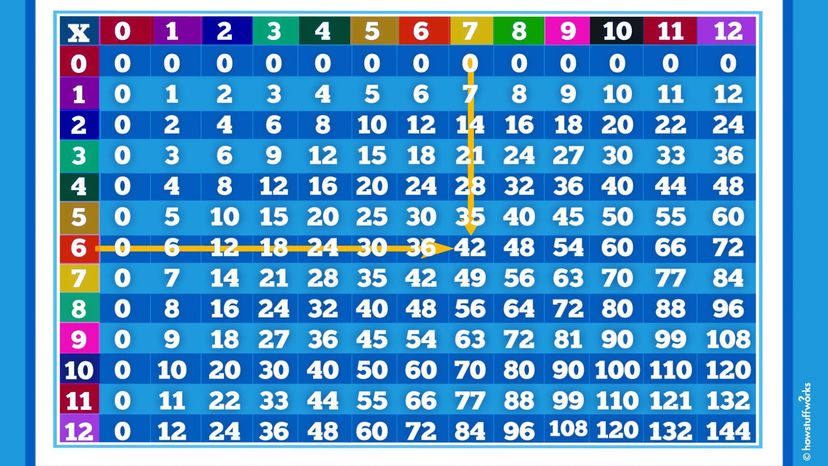# How to Use a Times Table (It's Not Magic, It's Memorization)

By: Kristen Hall-Geisler & Austin Henderson  |You want to multiply 7 x 6. So you find 7 in the top row and 6 on the left side. Follow the yellow arrow down from 7 and across from 6. They meet at 42 and that is your answer. HowStuffWorks

OK, here's the thing: Math isn't inherently miserable. Multiplication is kind of fun, and a multiplication table can help make it easier to learn. Whether you're a student, a teacher, or just someone fascinated by numbers, the times table has something for everyone.

Contents

## Understanding the Basics of the Times Table

Multiplication is the art of adding groups of things efficiently.

Imagine having three baskets of apples, with each basket holding four apples. Instead of counting each apple, you'd simply multiply 3 (baskets) by 4 (apples per basket) to get 12 (total apples). Not only is this method faster, but it also helps in understanding the concept of multiplication.

Now, to aid in this process, we use a multiplication chart known as the times table. This chart isn't just about multiplying two numbers; it's about recognizing patterns, using skip counting and memorizing for good practice.

For instance, pretend you want to multiply 7 by 6. Using the times table, you'd find 7 in a top row and 6 in a left column. Draw an imaginary line down from 7 and another one across from 6. Where they meet, you'd find your answer: 42.

## How to Efficiently Use the Table

Using the multiplication tables might seem like a puzzle at first, but with some good practice and patience, the times table becomes a handy tool for all your multiplication needs.

1. Finding patterns: Recognize patterns as you move through the rows and columns. For example, when you look at the multiples of 2, you'll notice they're all even numbers. This understanding of the pattern will make memorizing easier.
2. Memorizing chunks: Instead of trying to cram the entire multiplication table at once, break it into chunks. Start with the 1-12 tables, for instance. Take the "2 row," go across the numbers, and repeat the multiplication facts. "2 x 0 = 0, 2 x 1 = 2, 2 x 2 = 4..." and so on.
3. Skip counting: This is a method where you count by a particular number. For example, if you're learning the multiples of 3, you'd say, "3, 6, 9, 12..." This helps in memorizing the multiplication chart more effectively.

## The Advantages of Mastering the Times Table

While the process of learning and memorizing the times table might seem tedious to some, its benefits extend far beyond simple arithmetic. Here's why mastering the multiplication chart is invaluable.

1. Quick mental calculations: In everyday life, we constantly encounter situations where basic multiplication is required, be it while shopping, cooking or planning an event. Having the times table memorized means you can make these calculations rapidly without resorting to a calculator.
2. Foundation for advanced math: Understanding multiplication is fundamental to progressing in more complex mathematical areas such as algebra, calculus and even geometry. A strong grasp of the times table provides a sturdy foundation for these subjects.
3. Trains your brain: The process of memorizing and recalling multiplication facts sharpens the brain and enhances memory skills. This cognitive exercise can aid in other areas of learning and daily tasks.
4. Increased confidence in math: A student who has the multiplication chart at their fingertips often feels more confident in tackling math problems. This confidence can lead to a more positive attitude towards math and learning in general.
5. Real-world applications: From understanding interest rates to planning budgets, multiplication plays a role in numerous real-world scenarios. A strong grasp of the times table ensures you're equipped to handle these situations with ease.

This article was updated in conjunction with AI technology, then fact-checked and edited by a HowStuffWorks editor.